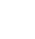# VBA Sort a range A-Z

Sorting a range of text from A-Z or Z-A is relatively simple in Excel, whcihever version you use. Select the range and click the A-Z icon to sort in the ribbon and follow any instructions.

However there may be times when you want to carry out this task in the middle of a VBA process or part of a database lookup system. We would never recommend sorting any database records A-Z as a matter of course but that is not to say that this shouldn’t be done. Datanology use this process in several projects, usually when a lookup list for a combobox is already unique and additions are occasionally mnade that need to be in some sort of alphabetical order.

The code example below actually uses the lastrow function as well, sorting data in Columns A-F as far down as the last used row in column A, where the data in column B needs to be in alphabetic order.

There are many different ways of sorting, and different rules you can apply, but this example is the most straightforward. You can record a Macro in Excel and copy the principal or parts of it to this code to adapt it to your own needs.

``` Public Sub AtoZ_by_Col_B() With Sheets("Data") lastrow = .Cells(.Rows.Count, "A").End(xlUp).Row End With Worksheets("Data").Select Columns("A:F").Select ActiveWorkbook.Worksheets("Data").Sort.SortFields.Clear ActiveWorkbook.Worksheets("Data").Sort.SortFields.Add Key:=Range("B2:B" & lastrow) _ , SortOn:=xlSortOnValues, Order:=xlAscending, DataOption:=xlSortNormal With ActiveWorkbook.Worksheets("Data").Sort .SetRange Range("A1:F" & lastrow) .Header = xlYes .MatchCase = False .Orientation = xlTopToBottom .SortMethod = xlPinYin .Apply End With End Sub ```